# Algebra II : Proportionalities

## Example Questions

1 2 3 5 Next →

### Example Question #11 : How To Find Inverse Variation

The number of days to construct a house varies inversely with the number of people constructing that house. If it takes 28 days to construct a house with 6 people helping out, how long will it take if 20 people are helping out?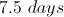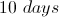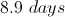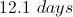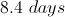Explanation:

The statement, 'The number of days to construct a house varies inversely with the number of people constructing that house' has the mathematical relationship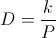, where D is the number of days, P is the number of people, and k is the variation constant. Given that the house can be completed in 28 days with 6 people, the k-value is calculated.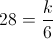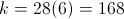This k-value can be used to find out how many days it takes to construct a house with 20 people (P = 20).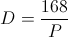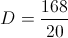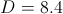So it will take 8.4 days to build a house with 20 people.

### Example Question #41 : Basic Single Variable Algebra

The speed of a turtle is indirectly proportional to its weight in pounds. At 10 pounds, the turtle's speed was 0.5.   What is the speed of the turtle if it grew and weigh 50 pounds?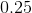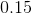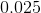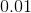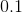Explanation:

Write the formula for the indirect proportional relationship.  If one variable increases, the other variable must also decrease.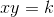Using speed and weight asand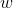respectively, the equation becomes: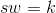Use the initial condition of the turtle's speed and weight to solve for theconstant.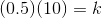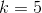Substitute this value back into the formula.  The formula becomes: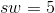We want to know the speed of the turtle when it is 50 pounds.  Divide the variableon both sides to isolate the speed variable.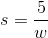Substitute the new weight of the turtle.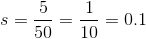### Example Question #104 : Mathematical Relationships

x varies inversely with y. When x=10, y=6. When x=3, what is y?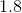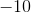Explanation:

Inverse variation takes the form: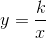Plugging in: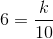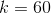Then solve when x=3: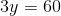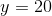### Example Question #105 : Mathematical Relationships

The rate of speed is indirectly proportional to the time it takes to travel somewhere. If a person walks at a rate of 3 miles per hour and it takes them 4 hours to get to their destination how far did they travel?Explanation:

For an indirect proportionality, use the equationIf rate of speed is indirectly proportional to the time, then the speed is y (3 miles per hour) and the time is x (4 hours).

Replace those into the indirect proportionality formula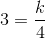Solve for k by multiplying by 4 on both sides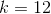The person traveled 12 miles.

### Example Question #21 : Indirect Proportionality

A turtle moves at a pace that is inversely proportional to its weight. Suppose at five pounds it moves two feet per minute. What would be the turtle's speed in feet per minute if it weighed eight pounds?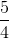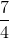Explanation:

Write the relationship for inverse proportionality.  If one variable increases, the other variable must decrease.orLetbe the turtle's speed, andis the weight of the turtle since the turtle's speed depends on its weight.

Solve forby substituting the initial conditions.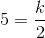Multiply by two on both sides.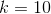Substitute this value back into the original equation.

The relationship is: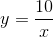Substitute eight pounds to find its speed.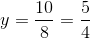The turtle will move at a rate offeet per minute.

1 2 3 5 Next →

### All Algebra II Resources